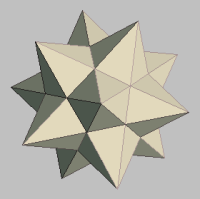MATHGOTSERVED# Interactive Practice Tests

ARITHMETIC
ELEMENTARY ALGEBRA
COLLEGE ALGEBRA
Welcome to the Accuplacer Math Test Preparation center. Here you will find a wealth of resources that will increase your odds of passing the Accuplacer Math Placement test. There are three sections for the Accuplacer Math test series which are 1) Arithmetic 2) Elementary Algebra, and 3) College Level Mathematics. Below is a collection of links that will help you prepare for the Accuplacer math test.
Please wait for the test to load below....
ARITHMETIC
ELEMENTARY ALGEBRA
COLLEGE ALGEBRA